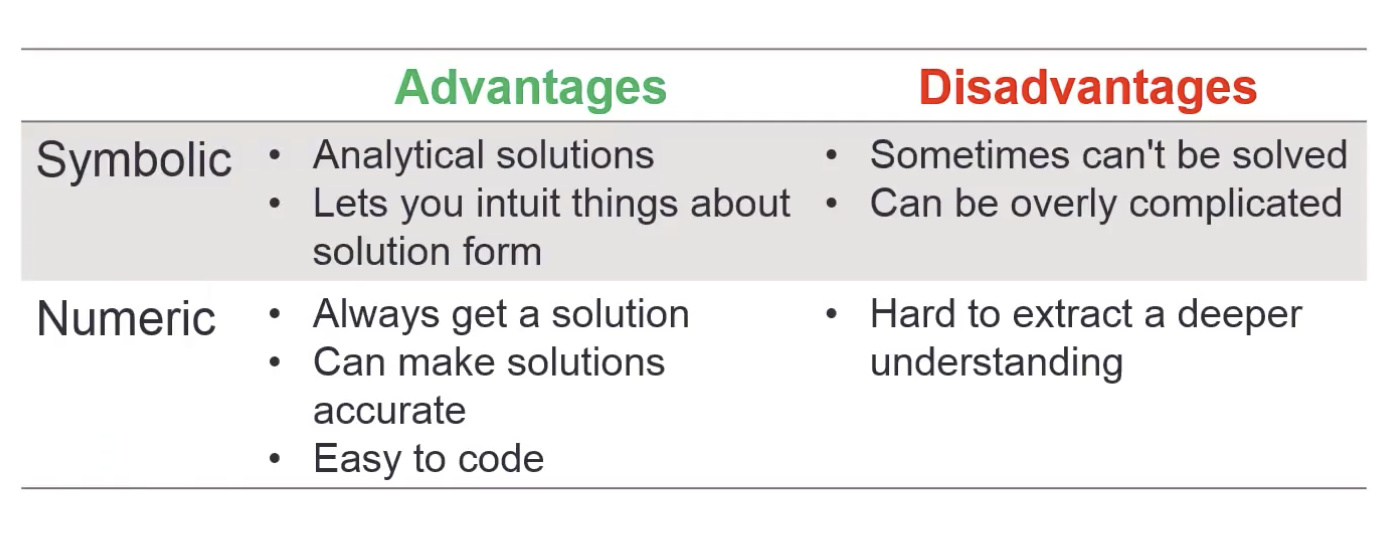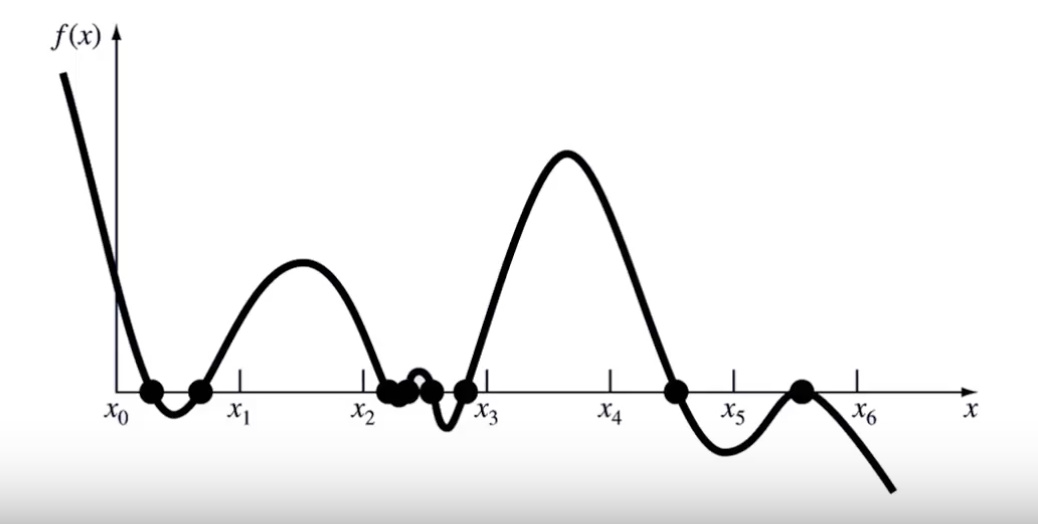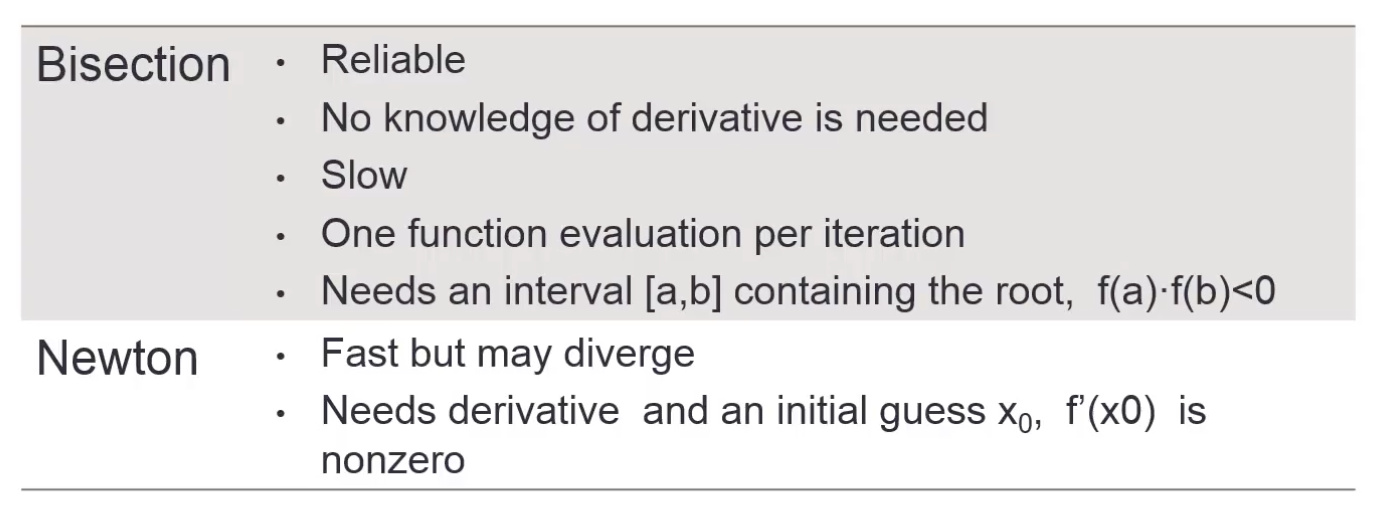# MATLAB 求方程的根——台大郭彦甫学习笔记

#### Problem Statement

• Suppose you have a mathematical function f ( x ) f(x) and you want to find x 0 x_0 such that f ( x o ) = 0 f(x_o)=0 , e.g.

f ( x ) = x 2 − 2 x − 8 = 0 f(x) = x^2 - 2x - 8 = 0

• Solution1:Analytical Solution

• Solution2:Graphical Illustration

• Solution3:Numerical Solution

#### Symbolic Root Finding Approach

• Performing mathematics on symbols, NOT numbers

• The symbols math are performed using “symbolic variables”

• Use sym or symsto create symbolic variables.

syms x
% or x = sym('x');
x + x + x


#### Symbolic Root Finding:solve()

• Function solvefinds roots for equations

y = x ⋅ s i n ( x ) − x = 0 y = x\cdot{sin(x)} - x = 0

syms x
y = x*sin(x)-x;
solve(y,x)
%solve('x*sin(x)-x',x)


#### Solving Multiple Equations

• Solve this equation using symbolic approach:

{ x − 2 y = 5 x + y = 6 \begin{cases} x-2y= 5\\ x+y= 6 \end{cases}

syms x y
eq1 = x-2*y-5;
eq2 = x+y-6;
A = solve(eq1,eq2,x,y)


#### Solving Equations Expressed in Symbols

• What if we are given a function expressed in symbols?

a x 2 − b = 0 ax^2-b=0

syms x a b
solve('a*x^2-b')

• x x is always the first choice to be solved

• What if one wants to express b b in tems of a a and x x ?

syms x a b
solve('a*x^2-b','b')


#### Exercise

• Solve this equation for x x using symbolic approach

( x − 1 ) 2 + ( y − b ) 2 = r 2 (x-1)^2 + (y-b)^2 = r^2

syms x y a b r
solve((x-a)^2+(y-b)^2 - r^2)

• Find the matrix inverse using symbolic approach

[ a b c d ] \begin{bmatrix} a &amp; b\\ c &amp; d \end{bmatrix}

syms a b c d
A = [a b; c d];
B = inv(A)


#### Symbolic Differentiation : diff()

• Caculate the derivative of a symbolic function:

y = 4 x 5 y = 4x^5

syms x
y = 4*x^5;
yprime = diff(y)

• Exercise:

f ( x ) = e x 2 x 3 − x + 3 , d f d x = ? f(x) = \frac{e^{x^2}}{x^3-x+3} , \frac{df}{dx}=?

syms x
f = exp(x^2)/(x^3-x+3);
fprime = diff(f)


f ( x ) = x 2 + x y − 1 y 3 + x + 3 , ∂ f ∂ x = ? f(x) = \frac{x^2+xy-1}{y^3+x+3} , \frac{\partial f}{\partial x}=?

syms x y
f = (x^2+x*y-1)/(y^3+x+3);
fprime = diff(f)


#### Symbolic Integration : int()

• Calculate the integral of a symbolic function:

z = ∫ y d x = ∫ x 2 e x d x , z ( 0 ) = 0 z = \int ydx = \int x^2e^xdx , z(0)=0

syms x;
y = x^2*exp(x);
z = int(y);
z = z-subs(z,x,0)


#### Exercise:

∫ 0 10 x 2 − x + 1 x + 3 d x \int_0^{10}\frac{x^2-x+1}{x+3}dx

syms x;
y = (x^2-x+1)/(x+3);
z = int(y,0,10)


#### Symbolic vs Numeric#### Review of Function Handles(@)

• A handle is a pointer to a function

• Can be used to pass functions to other functions

• For example, the input of the following function is another function:

function [y] = xy_plot(input,x)  % input is a function variable
% xy_plot receives the handle of a function and plots that
% function of x
y = input(x);
plot(x,y,'r--');
xlabel('x');
ylabel('function(x)');
end

• When we need to use the function xy_plot ,use xy_plot(@sin,0:0.01:2*pi);

#### fsolve()

• A numeric root solver

• For example, solve this equation:

f ( x ) = 1.2 x + 0.3 + x ⋅ s i n ( x ) f(x) = 1.2x+0.3+x\cdot sin(x)

f2 = @(x) (1.2*x+0.3+x*sin(x));  % inline function
fsolve(f2,0)  % f2 is a function handle ; 0 is a initial guess


#### Exercise

• Find the root for this equation:

f ( x , y ) = { 2 x − y − e − x − x + 2 y − e − y f(x,y)=\begin{cases}2x-y-e^{-x} \\ -x+2y-e^{-y}\end{cases}

• using initial value(x,y) = (-5,5)

#### fzero()

• Another numeric root solver

• Find the zero if and only if the function crosses the x-axis

f = @(x) x.^2
fzero(f,0.1)  % 0.1 is a initial guess
fsolve(f,0)

• Options:

f = @(x) x.^2
options = optimset('MaxIter',le3,'TolFun',1e-10);  % 1e3 is 'Number of iterations'; 1e-10 is Tolerance(误差)
fsolve(f,0.1,options)
fzeros(f,0.1,options)


#### Finding Roots of Polynomials : roots()

• Find the roots of this polynomial:

f ( x ) = x 5 − 3.5 x 4 + 2.75 x 3 + 2.125 x 2 − 3.875 x + 1.25 f(x) =x^5-3.5x^4+2.75x^3+2.125x^2-3.875x+1.25

roots([1 -3.5 2.75 2.125 -3.875 1.25])

• roots only works for polynomials

### How Do These Solvers Find the Roots?

• Now we are going to introduce more details of some numeric methods#### Numeric Root Finding Methods

• Two major types:

• Bracketing methods(3.g.,bisection method)
• Open methods(3.g.,Newton-Raphson method)
• Roots are found iteratively until some criteria are satisfied:

• Accuracy
• Number of iteration
• Bisection vs Newton09-20
12-0611-30355
08-215万+
08-142083
07-207316
08-073232
06-11
12-15
05-22
07-22930
05-211万+
08-191472
04-265901
11-074485点击重新获取扫码支付余额充值